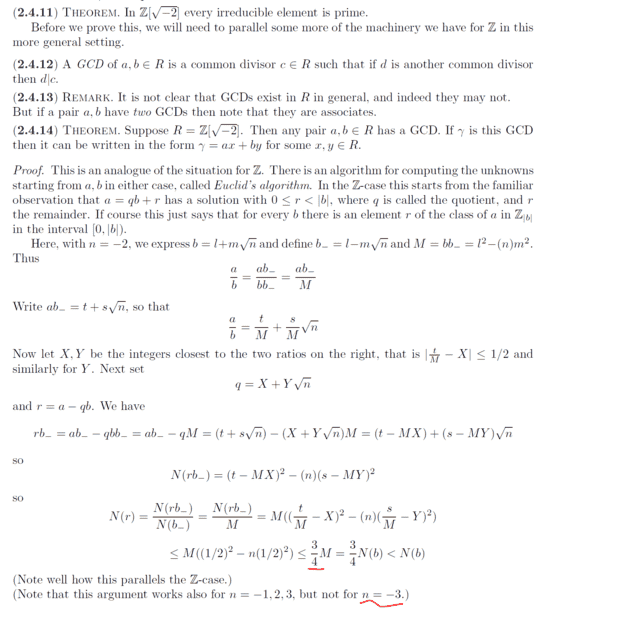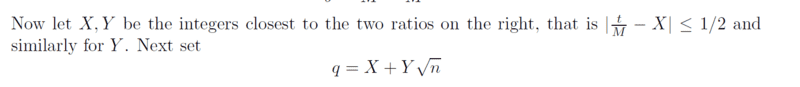# Problem involving irreducible element -Ring theory

Gold Member
Homework Statement:
see attached...
Relevant Equations:
Ring theory
Hey guys, i need insight on the highlighted part...the steps before this are quite clear:where is the ##\dfrac{3}{4}M ## coming from? and how to show the argument does not work for ##n=-3##? i should be able to check on this ...later.

and why is the Norm of say;

##ϒ =a+c\sqrt b##

taken as

##N(ϒ)=a^2-c^2b##

and not

##N(ϒ)=a^2+c^2b##?

cheers

Homework Helper
2022 Award
In $\mathbb{Q}[X : X^2 \in \mathbb{Q}] \supset \mathbb{Z}[X]$ we have $$(a + bX)(a - bX) = a^2 - b^2X^2 \in \mathbb{Q}.$$ If this is zero, then we have either $a + bX = 0$ or $a - bX = 0$ and in either case $a = b = 0$. Hence for $a + bX \neq 0$ we have $$(a +bX)^{-1} = \frac{a - bX}{a^2 - b^2X^2}.$$ Note that if $X^2 = - N < 0$, as in your case, then $$a^2 - b^2 X^2 = a^2 + Nb^2.$$

•chwala
Gold Member
Homework Statement:: see attached...
Relevant Equations:: Ring theory

Hey guys, i need insight on the highlighted part...the steps before this are quite clear:

View attachment 323675

where is the ##\dfrac{3}{4}M ## coming from? and how to show the argument does not work for ##n=-3##? i should be able to check on this ...later.

and why is the Norm of say;

##ϒ =a+c\sqrt b##

taken as

##N(ϒ)=a^2-c^2b##

and not

##N(ϒ)=a^2+c^2b##?

cheers
...
I am trying to follow this with a practical example...i still have doubts on the highlighted, let us consider; the gcd ##(7,4)## with ##n=-2## for example, then we shall have (using Euclid algorithm),

##7=1⋅4+3##

...
##\dfrac{7}{4}= \dfrac{7(l-m\sqrt{-2})}{l^2+2m^2}=\dfrac{7l}{l^2+2m^2}-\dfrac{m\sqrt{-2}}{l^2+2m^2}##

that is by following the attached literature... then

##\dfrac{t}{M}=\dfrac{7l}{l^2+2m^2}##

and

##\dfrac{s\sqrt{n}}{M}=\dfrac{m\sqrt{-2}}{l^2+2m^2}##

...let ##X, Y## be the closest integers to the two ratios on the right...not quite understanding this statement...do they mean ##X## an integer value i.e close to ##\dfrac{7l}{l^2+2m^2}## and ##Y## an integer value close to ##\dfrac{m\sqrt{-2}}{l^2+2m^2}##?

Gold Member
...
I am trying to follow this with a practical example...i still have doubts on the highlighted, let us consider; the gcd ##(7,4)## with ##n=-2## for example, then we shall have (using Euclid algorithm),

##7=1⋅4+3##

...
##\dfrac{7}{4}= \dfrac{7(l-m\sqrt{-2})}{l^2+2m^2}=\dfrac{7l}{l^2+2m^2}-\dfrac{m\sqrt{-2}}{l^2+2m^2}##

that is by following the attached literature... then

##\dfrac{t}{M}=\dfrac{7l}{l^2+2m^2}##

and

##\dfrac{s\sqrt{n}}{M}=\dfrac{m\sqrt{-2}}{l^2+2m^2}##

...let ##X, Y## be the closest integers to the two ratios on the right...not quite understanding this statement...do they mean ##X## an integer value i.e close to ##\dfrac{7l}{l^2+2m^2}## and ##Y## an integer value close to ##\dfrac{m\sqrt{-2}}{l^2+2m^2}##?
I think i now understand this part;It basically means;

##|\frac{t}{M} - X|≤\frac{1}{2}##

and

##|\frac{s\sqrt {n}}{M} - Y|≤\frac{1}{2}##

i.e

##-\frac{1}{2}≤\frac{t}{M} - X≤\frac{1}{2}##

and

##-\frac{1}{2}≤\frac{s\sqrt{n}}{M} - Y≤\frac{1}{2}##

it is true that, (on adding them)

##0≤\frac{t}{M}+\frac{s\sqrt{n}}{M}- X-Y≤1##

Staff Emeritus
Homework Helper
Gold Member
Homework Statement:: see attached...
Relevant Equations:: Ring theory

Hey guys, i need insight on the highlighted part...the steps before this are quite clear:

View attachment 323675

where is the ##\dfrac{3}{4}M ## coming from? and how to show the argument does not work for ##n=-3##? i should be able to check on this ...later.

cheers
The proof uses an arbitrary value for the integer, ##n##, through the point where

##\displaystyle N(r) \le M( (1/2)^2-(n)(1/2)^2)## .

Then substituting ##\displaystyle n=-2## one sees that ##\displaystyle M( (1/2)^2-(-2)(1/2)^2)=\dfrac{3}{4}M<N(b)## .

What do you get for ##n=-3## ?

Last edited:
Staff Emeritus
Homework Helper
Gold Member
...
I am trying to follow this with a practical example...i still have doubts on the highlighted, let us consider; the gcd ##(7,4)## with ##n=-2## for example, then we shall have (using Euclid algorithm),

##7=1⋅4+3##

...
##\dfrac{7}{4}= \dfrac{7(l-m\sqrt{-2})}{l^2+2m^2}=\dfrac{7l}{l^2+2m^2}-\dfrac{m\sqrt{-2}}{l^2+2m^2}##

that is by following the attached literature... then

##\dfrac{t}{M}=\dfrac{7l}{l^2+2m^2}##

and

##\dfrac{s\sqrt{n}}{M}=\dfrac{m\sqrt{-2}}{l^2+2m^2}##

...let ##X, Y## be the closest integers to the two ratios on the right...not quite understanding this statement...do they mean ##X## an integer value i.e close to ##\dfrac{7l}{l^2+2m^2}## and ##Y## an integer value close to ##\dfrac{m\sqrt{-2}}{l^2+2m^2}##?
This is not a particularly good example of two elements in ##\displaystyle \mathbb{Z} [\sqrt{-2\,} \,] ## .

Writing them as elements of ##\displaystyle \mathbb{Z} [\sqrt{-2\,} \,] ## , we have ##\displaystyle 7= 7+0\sqrt{-2\,}## , and ##\displaystyle 4= 4+0\sqrt{-2\,}##

So, we have ##l=4\,,\ m=0\,,\ \text{ and } M=16## .

This gives ##\displaystyle \dfrac{t}{M}=\dfrac{7l}{M}=\dfrac{28}{16}\, ,## which reduces to ##\displaystyle \dfrac 7 4 = 1.75## . And for ##\displaystyle \dfrac{s\sqrt{-2\,}}{M}## we have ##\displaystyle \dfrac{m\sqrt{-2\,}}{M}=0 ## .

Being the integer closest to ##1.75##, we have ##X=2## . It should be apparent that ##Y=0## . This gives ##\displaystyle q= X+Y\sqrt{-2\,}=2+0\,\sqrt{-2\,}=2##

Therefore, ##\displaystyle \ r = a-q\,b = 7-2\cdot 4 =-1##.

This is very similar to the standard version of Euclidean Division, except that here the remainder falls in the interval ##\displaystyle [-b/2 \,, \ b/2]\, ##. (The author should clean that up to make one of the ends of the interval be open.)

The Euclidean Algorithm goes further than this. The next step: Promote ##b## to take the role of ##a## and ##r## to take the role of ##b##, i.e. - divide ##4## by ##-1## find a quotient and a remainder.. If the remainder is zero, you are nearly (or half) done. If the new ##r\ne 0##, promote ##(b,\ r)## to ##(a,\ b)## and repeat . . . until some ##r=0## . The previous value of ##r## is the ##\gcd (a,\ b)## .

The final step in the Euclidean Algorithm has you backtrack through your previous steps to express the gdc as ##\displaystyle \gcd (a,\ b)=ua+vb ## for some ##u## and ##v##.

•chwala
Gold Member
This is not a particularly good example of two elements in ##\displaystyle \mathbb{Z} [\sqrt{-2\,} \,] ## .

Writing them as elements of ##\displaystyle \mathbb{Z} [\sqrt{-2\,} \,] ## , we have ##\displaystyle 7= 7+0\sqrt{-2\,}## , and ##\displaystyle 4= 4+0\sqrt{-2\,}##

So, we have ##l=4\,,\ m=0\,,\ \text{ and } M=16## .

This gives ##\displaystyle \dfrac{t}{M}=\dfrac{7l}{M}=\dfrac{28}{16}\, ,## which reduces to ##\displaystyle \dfrac 7 4 = 1.75## . And for ##\displaystyle \dfrac{s\sqrt{-2\,}}{M}## we have ##\displaystyle \dfrac{m\sqrt{-2\,}}{M}=0 ## .

Being the integer closest to ##1.75##, we have ##X=2## . It should be apparent that ##Y=0## . This gives ##\displaystyle q= X+Y\sqrt{-2\,}=2+0\,\sqrt{-2\,}=2##

Therefore, ##\displaystyle \ r = a-q\,b = 7-2\cdot 4 =-1##.

This is very similar to the standard version of Euclidean Division, except that here the remainder falls in the interval ##\displaystyle [-b/2 \,, \ b/2]\, ##. (The author should clean that up to make one of the ends of the interval be open.)

The Euclidean Algorithm goes further than this. The next step: Promote ##b## to take the role of ##a## and ##r## to take the role of ##b##, i.e. - divide ##4## by ##-1## find a quotient and a remainder.. If the remainder is zero, you are nearly (or half) done. If the new ##r\ne 0##, promote ##(b,\ r)## to ##(a,\ b)## and repeat . . . until some ##r=0## . The previous value of ##r## is the ##\gcd (a,\ b)## .

The final step in the Euclidean Algorithm has you backtrack through your previous steps to express the gdc as ##\displaystyle \gcd (a,\ b)=ua+vb ## for some ##u## and ##v##.
Thanks @SammyS ...i will go through your remarks later...cheers.